Basic Mathematics

# Angles and Lines - Problem Solving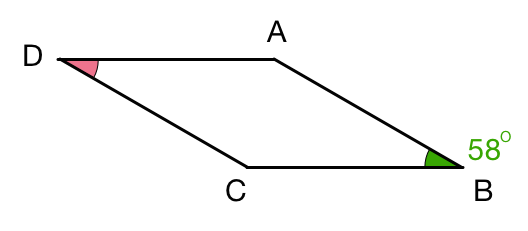In parallelogram $ABCD$, the green angle $\angle ABC = 58 ^ \circ$. What is the pink angle $\angle ADC$?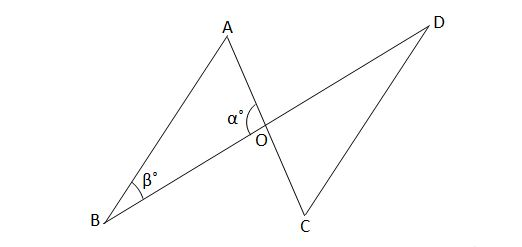In the above diagram, line segment $\overline{AB}$ is parallel to line segment $\overline{CD}.$ If $\alpha={70}^\circ$ and $\beta={28}^\circ,$ then what is the angle $\angle OCD ?$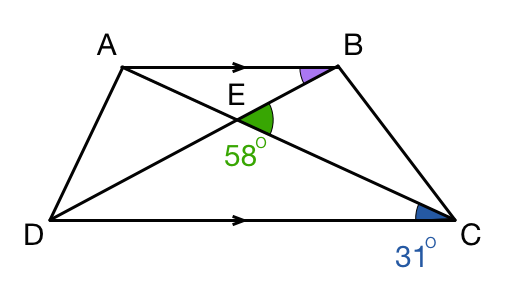Given quadrilateral $ABCD$ where $AB$ is parallel to $DC$ and $AC$ and $BD$ intersect at $E$, with $\angle BEC = 58 ^\circ$ and $\angle ACD = 31 ^\circ$, what is $\angle ABE$ (in degrees)?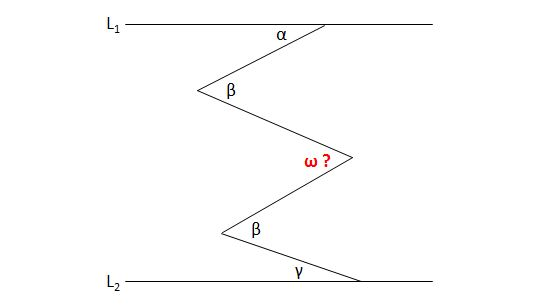$L_1$ is parallel to $L_2$ and $\alpha={54}^\circ,$ $\beta={84}^\circ$ and $\gamma={21}^\circ.$ What is the angle $\omega$ in degrees?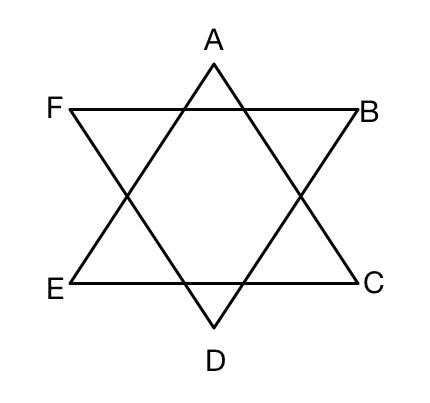Consider a 6 sided star that is formed from 2 triangles as above. What is the sum of the angles at A, B, C, D, E and F?

×